Real-time Personalization using Embeddings for Search Ranking at Airbnb 是 KDD 2018 的 best paper, 整篇文章读下来，初看好像只是套了 word2vec 来生成 user embedding 和 item embedding；但是细读下来，会发现其中有不少细节值得考究，这种风格跟 youtube 在 2016 年发表的那篇 Deep Neural Networks for YouTube Recommendations 很像，两篇都是实践性很强的 paper，非常值得看。而且两篇文章分别代表着 deep learning 中生成 embedding 的两大流派：无监督和有监督。本文主要讲的是 Airbnb 的这篇 paper 的基本做法和一些细节。

Airbnb 的这项工作的业务背景是：用户在其租房 app 上搜索时，需要返回一个 list 的推荐结果,在 paper 中提到了 Airbnb 用的是业界比较主流的 Learning To Rank 技术，关于 LTR 的技术细节可参考微软的 From RankNet to LambdaRank to LambdaMART: An Overview，Airbnb 用的也是 pairwise 模式下的 LambdaRank，而这篇 paper 主要描述的是如何通过 word2vec 的方式生成 user embedding 和 item embedding 作为 feature 供排序模型使用（文章中也将 item 称为 listing，所以后文中这两个词的含义是一样的）。

1. 将正反馈（用户最终下单，作为 global context）和负反馈（商家拒接接单，作为额外的一个data set）的信号加入到 skip-gram 中进行训练
2. 做 negative sampling 时，考虑到具体业务不仅仅在全集上做负采样
3. 同一个 uid 的样本较为稀疏，因此 user embedding 从 uid 粒度变为 user type 粒度，避免样本过于稀疏 embedding 学习不充分

## 离线训练

paper 中的离线训练可分为两大部分：short-term interest 和 long-term interest

### listing embedding

$$L=\sum_{s \in S}\sum_{l_i \in s}(\sum_{-m \le j \le m,j \ne 0}^M \log p(l_{i+j}|l_i))\tag{1}$$

$$p(l_{i+j}|l_i) = \frac{\exp(v_{l_j}^T v_{l_{i+j}}^{‘})}{\sum_{l=1}^{|V|}\exp(v_{l_i}^Tv_{l}^{‘})}\tag{2}$$

W 和 W’；具体可参考讲义 CS224n: Natural Language Processing with Deep Learning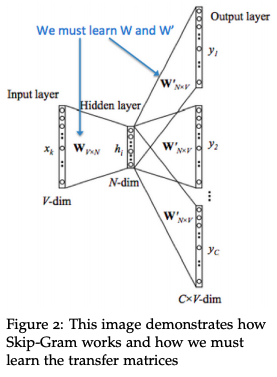$$\arg\max_{\theta} \sum_{(l,c) \in D_p} \log\frac{1}{1+e^{-v_{c}^{‘}v_l}} + \sum_{(l,c) \in D_n} \log\frac{1}{1+e^{v_{c}^{‘}v_l}} \tag{3}$$

(1)利用监督信号作为 global context

$$\arg\max_{\theta} \sum_{(l,c) \in D_p} \log\frac{1}{1+e^{-v_{c}^{‘}v_l}} + \sum_{(l,c) \in D_n} \log\frac{1}{1+e^{v_{c}^{‘}v_l}} + \\ \log \frac{1}{1+e^{-v_{l_b}v_l}}\tag{4}$$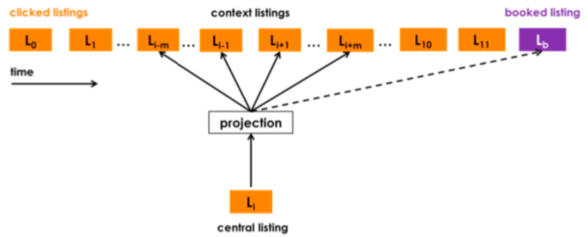(2)控制 negative sampling 的采样空间

$$\arg\max_{\theta} \sum_{(l,c) \in D_p} \log\frac{1}{1+e^{-v_{c}^{‘}v_l}} + \sum_{(l,c) \in D_p} \log\frac{1}{1+e^{v_{c}^{‘}v_l}} + \\ \log \frac{1}{1+e^{-v_{l_b}v_l}}+\sum_{(l,m_n) \in D_{m_n}} \log\frac{1}{1+e^{v_{m_n}^{‘}v_l}}\tag{5}$$

(3)冷启动的 item embedding

### user_type/listing_type embeddings

1. 数据更为稀疏，前面的是点击事件，这里则是转化事件
2. 某些用户的 booked listing 的长度可能只有 1，这样没法直接用 skip-gram 来训练
3. 对于每个 entity, 如果需要较充分地学习出其 embedding，每个 entity 至少要出现 5-10 次，而实际上不少 listing 被下单的次数是没有 5 次的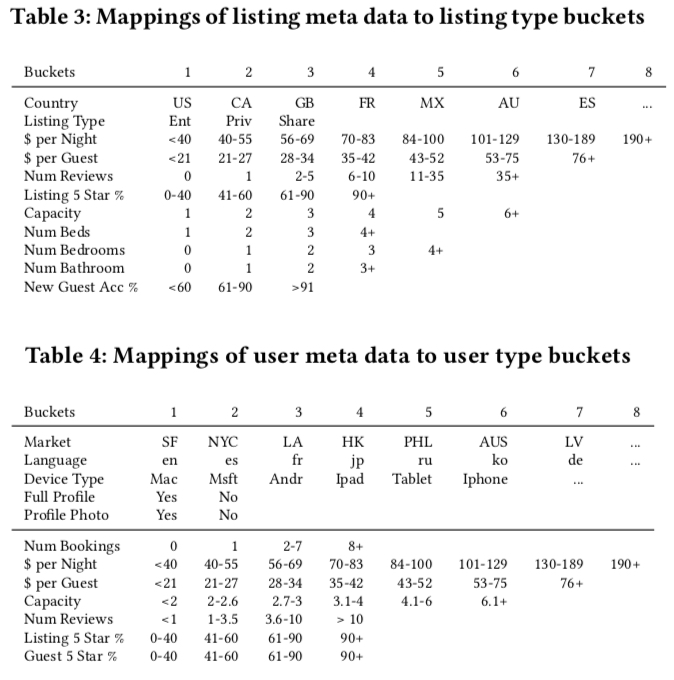paper 中解决这个问题的方法也和直接，就是将前面提到的每个 user 的booked listing $s_b=(l_{b1},l_{b2}…l_{bM}) \in S$ 变为 $s_b=(u_{type1},l_{type1}…u_{typeM}, l_{typeM}) \in S$, 值得注意的是，虽然每个 session 的 sequence 表示的是同一个user_id，但是其 user_type 是会随时间变化而变化的，但是 paper 中并没有提到改变 user_type 的时间窗口，猜测是一旦发生变化就改变，然后插入到上面的 booked listing 对应的时间位置上，（这里有个问题，这样做似乎是没法解决那些 booked listing 长度为 1 的数据）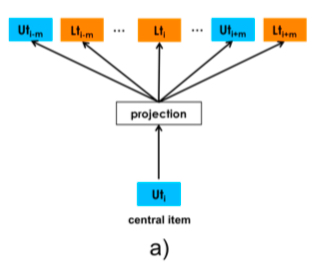$$\arg\max_{\theta} \sum_{(u_t,c) \in D_{book}} \log\frac{1}{1+e^{-v_{c}^{‘}v_{u_t}}} + \sum_{(u_t,c) \in D_{neg}} \log\frac{1}{1+e^{v_{c}^{‘}v_{u_t}}} \tag{6}$$

$$\arg\max_{\theta} \sum_{(u_t,c) \in D_{book}} \log\frac{1}{1+e^{-v_{c}^{‘}v_{u_t}}} + \sum_{(u_t,c) \in D_{neg}} \log\frac{1}{1+e^{v_{c}^{‘}v_{u_t}}} + \\ \sum_{(u_t,l_t) \in D_{neg}} \log\frac{1}{1+e^{v_{l_t}v_{u_t}}} \tag{7}$$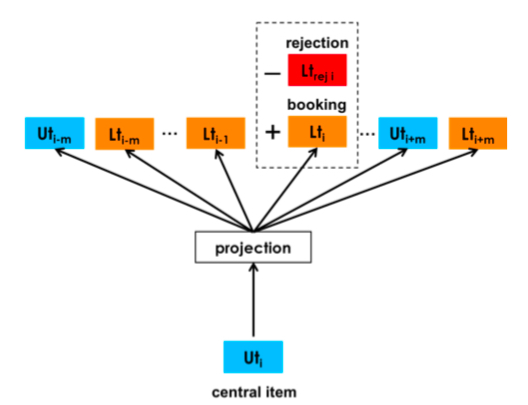### 训练细节

• 如前文所说，用了 800 million 的 click session，划分的标准就是同一个用户的连续两次点击如果超过 30min 就划分为两个 session，
• 去掉了一些无效点击（定义为点击后在页面停留时长小于 30s 的）
• 对于 booked session 做了 5 倍的 upsampling

• 天级更新，按照时间滑动窗口从当前时间往前取几个月的训练数据
• 每天都重新训练 listing embedding（随机初始化），而不是在之前训练好的基础上做 finetune，paper 称这样的效果比做 finetune 的要好
• embedding 的维度是 32（做了效果和资源的 trade-off），skipgram 的时间窗口是 5，训练 10 个 iteration 的效果是最好的

### embedding 有效性校验

embedding 训练出来后，怎么判断其有效性是个值得探讨的问题，尤其是在深度学习缺乏可解释性的情况下，paper 中用到了以下几种方法，都比较值得借鉴

(1) 可视化
(2) 计算 listing embedding 之间的cosine similarity
(3) 根据 embedding similarity 对历史日志中的 item 重新排序，看 booked item 的位置（越小越好）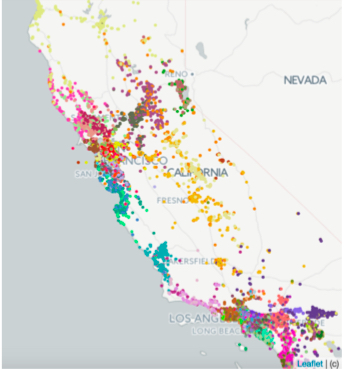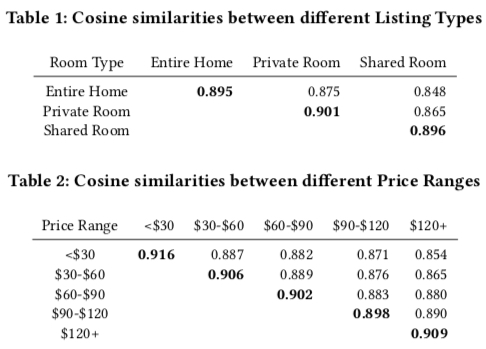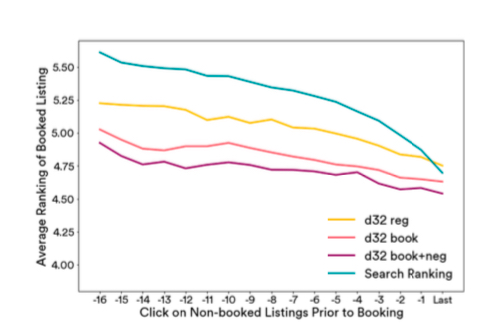• search ranking：排序的后验，即历史 search session 的真正排序
• d32: 公式(3) 训练出来的 embedding, 即原始的 word2vec 方法
• d32 book: 公式(4) 训练出来的 embedding，即加上 booked item 作为 global context 训练出来的 embedding
• d32 book+neg: 公式(5)训练出来的 embedding，即做 nagative sampling 的时候专门考虑与 item 处于同一个 market 的 nagative samples

## 在线 serving

(1) Search Ranking: 用户搜索的排序模型
(2) Similar Listing Recommemdation：物品页面中相似物品动态的推荐

### Search Ranking

paper 中主要基于 embedding 间计算出来的相似性作为连续值特征，构造的特征列表如下图所示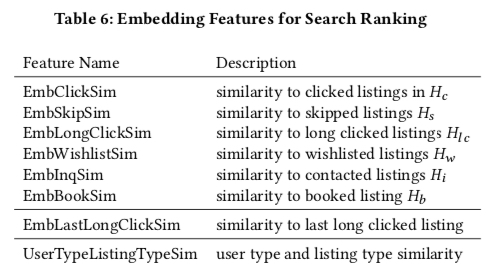• $H_c$: 用户过去两周点击过的 listing ids
• $H_{lc}$: 用户点击过且停留时长超过 60s 的 listing ids
• $H_s$: 用户点击的某个 listing 时跳过的其他的 listing ids（如点击了排序第 4 的，就跳过了前 3 个 listing）
• $H_w$: 用户过去两周添加到心愿单的 listing ids
• $H_i$: 用户过去两周联系过但是没有下单的 listing ids
• $H_b$: 用户过去两周下单过的 listing ids

$$EmbClickSim(l_c, H_c) = \max_{m \in M} cos(v_{l_i}, \sum_{l_h \in m, l_h \in H_c}v_{l_h})$$

• EmbClickSum 的重要性是这些 embedding 构造出来的 feature 中最重要的，反映了用户短期内的兴趣
• 描述下单的特征中，用户的长期兴趣比用户的短期兴趣更好（UserTypeListingTypeSim重要性高于 EmbBookSim），笔者猜测另一个原因可能是计算 EmbBookSim 数据会更为稀疏，因为一般对于一个用户来说两周内下单一次的频率是很低的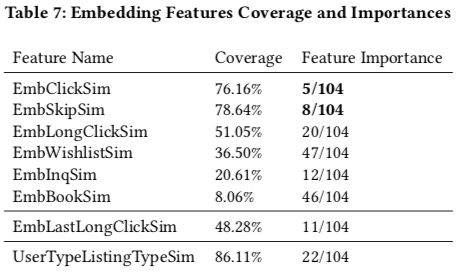### Similar Listing Recommemdation

Similar Listing Recommemdation 做的事情就是在每个 listing 详情页里推荐相似物品，这里主要利用的就是 listing embeddings 的 cos similarity 来选出 top-k 个最相似的物品， paper 将当前 airbnb 的相似物品推荐策略与这个基于 embedding 的方法作比较，线上的 AB 实验显示这个方法相比与当前的方法 ctr 提升了约 21%，cvr 提升了约 4.9%

## 小结

1. 如何训练生成 embedding
2. 怎么评估训练出来的 embedding 的有效性
3. 线上怎么使用这些embedding